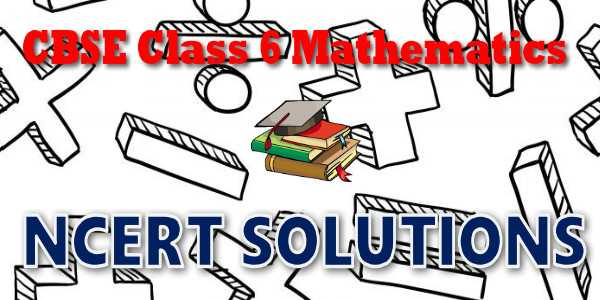No products in the cart.

# CBSE - Class 06 - Mathematics - Practical Geometry - NCERT Solutions## CBSE, JEE, NEET, NDA

Question Bank, Mock Tests, Exam Papers

NCERT Solutions, Sample Papers, Notes, Videos

## NCERT Solutions for Mathematics Practical Geometry## myCBSEguide App

Complete Guide for CBSE Students

NCERT Solutions, NCERT Exemplars, Revison Notes, Free Videos, CBSE Papers, MCQ Tests & more.

# NCERT solutions for class 6  Mathematics Practical Geometry## NCERT 6 Mathematics Text book Solutions

NCERT Class 6 Mathematics Chapter-wise Solutions

Maths Part I

• Chapter 1 - Knowing Our Numbers
• Chapter 2 - Whole Numbers
• Chapter 3 - Playing with Numbers
• Chapter 4 - Basic Geometrical Ideas
• Chapter 5 - Understanding Elementary Shapes
• Chapter 6 - Integers
• Chapter 7 - Fractions
• Chapter 8 - Decimals
• Chapter 9 - Data Handling
• Chapter 10 - Mensuration
• Chapter 11 - Algebra
• Chapter 12 - Ratio and Proportion
• Chapter 13 - Symmetry
• Chapter 14 - Practical Geometry

## CBSE class 6th Mathematics have one books. Book has chapters and topics.

• NCERT Mathematics Book Class 6

Here is the list of topics covered under each chapter of class 6 Mathematics NCERT text book.

• Exercise 1.1
• Exercise 1.2
• Exercise 1.3

• Exercise 2.1
• Exercise 2.2
• Exercise 2.3

• Exercise 3.1
• Exercise 3.2
• Exercise 3.3
• Exercise 3.4
• Exercise 3.5
• Exercise 3.6
• Exercise 3.7

• Exercise 4.1
• Exercise 4.2
• Exercise 4.3
• Exercise 4.4
• Exercise 4.5
• Exercise 4.6

• Exercise 5.1
• Exercise 5.2
• Exercise 5.3
• Exercise 5.4
• Exercise 5.5
• Exercise 5.6
• Exercise 5.7
• Exercise 5.8
• Exercise 5.9

• Exercise 6.1
• Exercise 6.2
• Exercise 6.3

• Exercise 7.1
• Exercise 7.2
• Exercise 7.3
• Exercise 7.4
• Exercise 7.5
• Exercise 7.6

• Exercise 8.1
• Exercise 8.2
• Exercise 8.3
• Exercise 8.4
• Exercise 8.5
• Exercise 8.6

• Exercise 9.1
• Exercise 9.2
• Exercise 9.3
• Exercise 9.4

### 10. Mensuration

• Exercise 10.1
• Exercise 10.2
• Exercise 10.3

### 11. Algebra

• Exercise 11.1
• Exercise 11.2
• Exercise 11.3
• Exercise 11.4
• Exercise 11.5

### 12. Ratio and Proportion

• Exercise 12.1
• Exercise 12.2
• Exercise 12.3

### 13. Symmetry

• Exercise 13.1
• Exercise 13.2
• Exercise 13.3

### 14. Practical Geometry

• Exercise 14.1
• Exercise 14.2
• Exercise 14.3
• Exercise 14.4
• Exercise 14.5
• Exercise 14.6

## NCERT Solutions Chapter 14: Practical Geometry

We see a number of shapes with which we are familiar. We also make a lot of pictures. These pictures include different shapes. We have learnt about some of these shapes in earlier chapters as well. Why don’t you list those shapes that you know about alongwith how they appear? In this chapter we shall learn to make these shapes. In making these shapes we need to use some tools. We shall begin with listing these tools, describing them and looking at how they are used.

We are going to consider “Ruler and compasses constructions”, using ruler, only to draw lines, and compasses, only to draw arcs. Be careful while doing these constructions. Here are some tips to help you.

• Draw thin lines and mark points lightly.
• Maintain instruments with sharp tips and fine edges.
• Have two pencils in the box, one for insertion into the compasses and the other to draw lines or curves and mark points.

## NCERT Solutions for Class 6th Mathematics

NCERT Solutions Class 6 Mathematics PDF (Download) Free from myCBSEguide app and myCBSEguide website. Ncert solution class 6 Mathematics includes text book solutions from  NCERT Solutions for CBSE Class 6 Mathematics have total 14 chapters. Class 6 Mathematics ncert Solutions in pdf for free Download are given in this website. Ncert Mathematics class 6 solutions PDF and Mathematics ncert class 6 PDF solutions with latest modifications and as per the latest CBSE syllabus are only available in myCBSEguide.

## NCERT Solutions for Class 5

NCERT Solutions for Class 4

## NCERT Solutions for Class 3## myCBSEguide

Trusted by 1 Crore+ Students

#### Question Paper Creator

• Create papers in minutes
• Print with your name & Logo